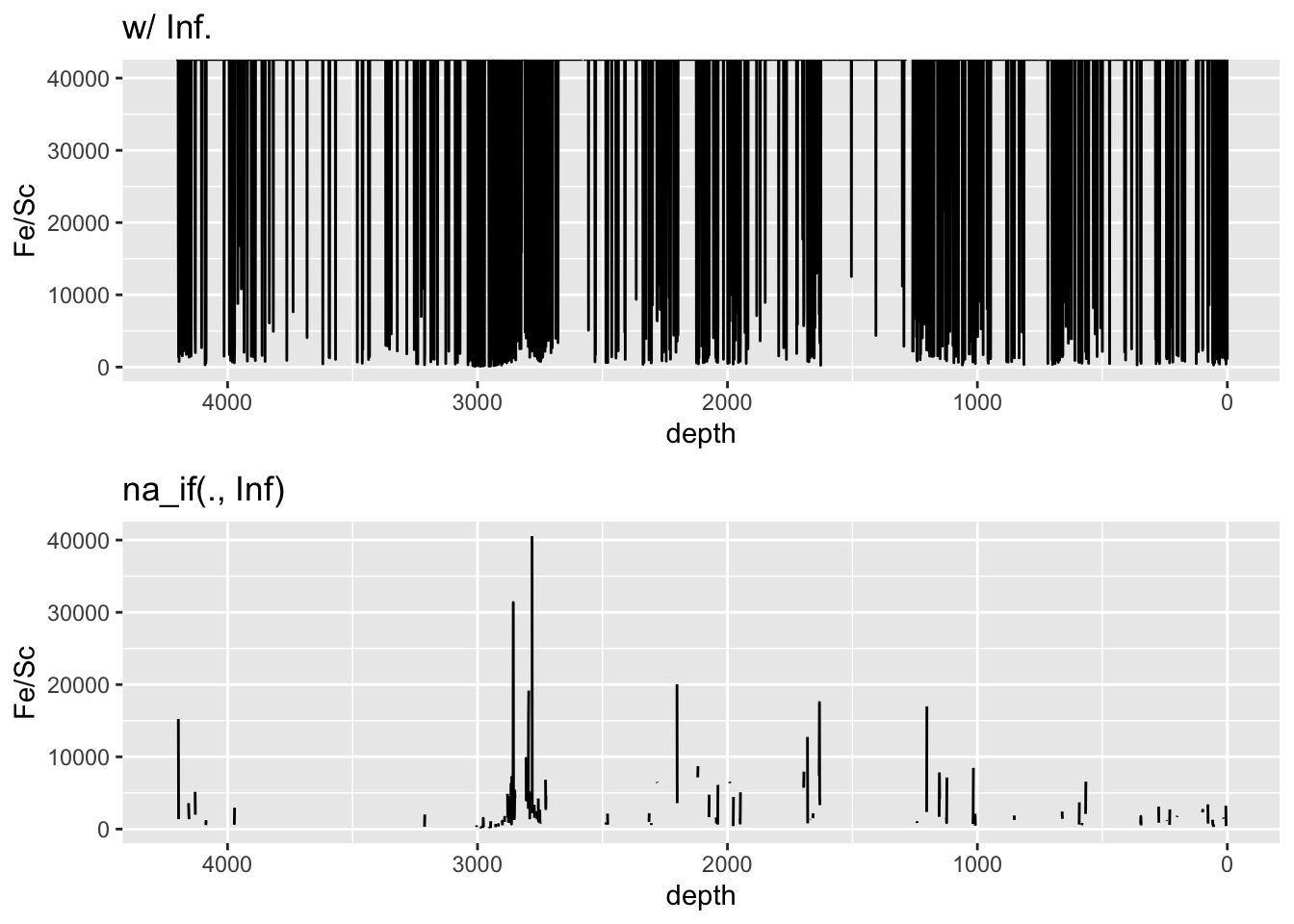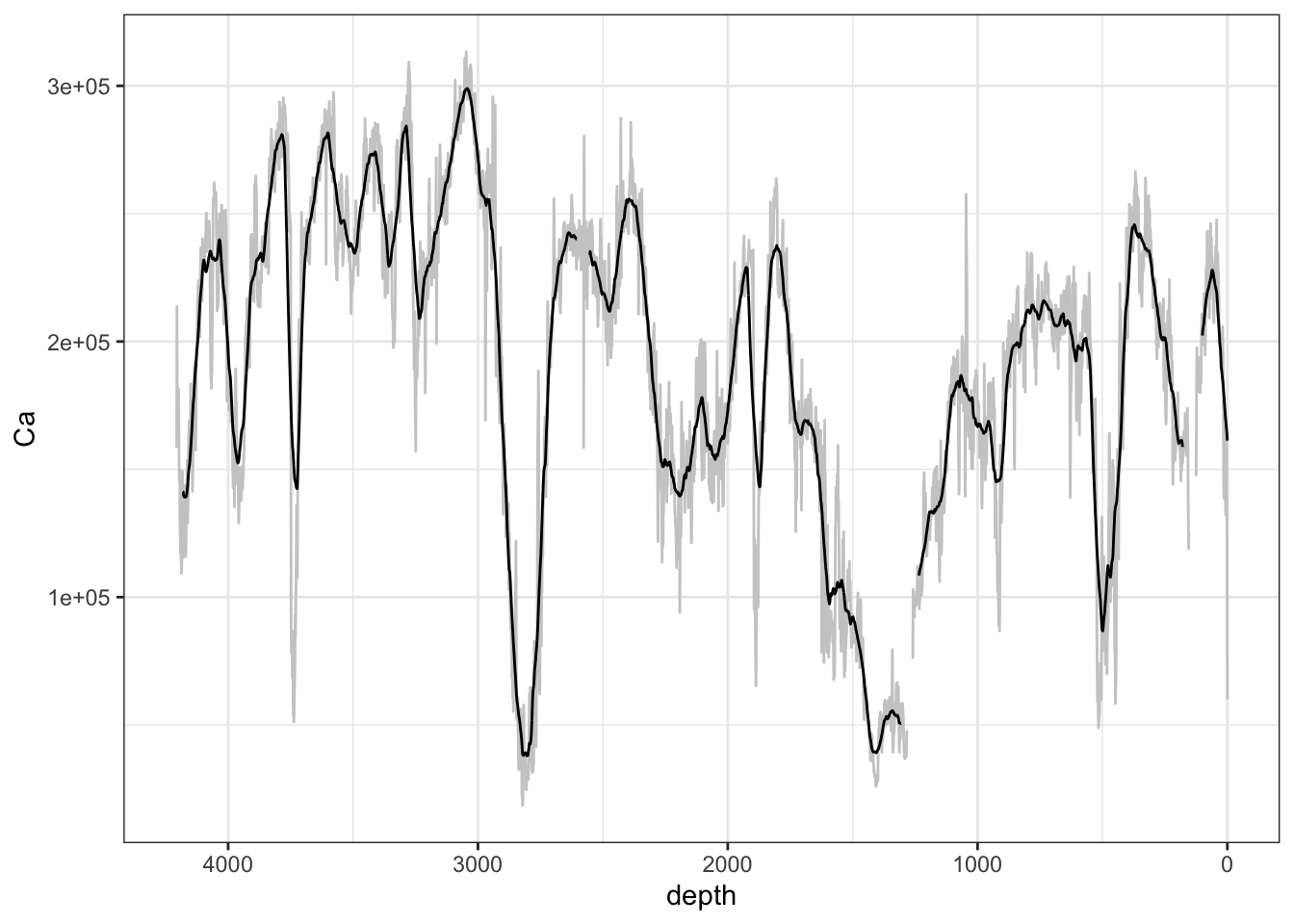## 6.2 Principal Component Analysis

Principal component analysis (PCA) is a common method for exploring multivariate data. Note the use of `zeroreplace()` - this is because the `princomp()` method defined for th `acomp` class uses a centred-log-ratio (`clr()`) transformation that is intolerant to zero-values.

``````CD166_19_xrf_acomp %>%
zeroreplace() %>%
princomp() %>%
biplot(xlabs = rep(".",times = nrow(CD166_19_xrf_acomp)))``````It is useful to plot components over depth. They can be extracted and plotted as follows:

``````bind_rows(
tibble(depth = CD166_19_xrf %>%
filter(qc == FALSE) %>%
pull("depth"),
Comp.1 = NA
),

tibble(
depth = CD166_19_xrf %>%
filter(qc == TRUE) %>%
pull("depth"),
Comp.1 = CD166_19_xrf_acomp %>%
zeroreplace() %>%
princomp() %>%
magrittr::extract2("scores") %>%
as_tibble() %>%
pull("Comp.1")
)) %>%

arrange(depth) %>%

ggplot(aes(x = depth, y = Comp.1)) +
geom_line() +
scale_x_reverse(name = "depth [mm]")``````
``## Warning: Removed 3 row(s) containing missing values (geom_path).``